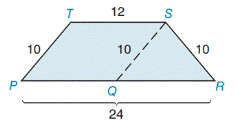Chapter 8.1, Problem 21EElementary Geometry For College St...

7th Edition
Alexander + 2 others
ISBN: 9781337614085

Solutions

Chapter
SectionElementary Geometry For College St...

7th Edition
Alexander + 2 others
ISBN: 9781337614085
Textbook Problem

In Exercises 19 to 22, find the area of the shaded region.▱ P Q S T

To determine

To find:

The area of the shaded region of the figure.

Explanation

1) Procedure:

Finding the area of the figure is followed by identifying the figure and find area with the suitable geometry formulae.

2) Calculation:

Given,

which represents the compound figure of a PQST parallelogram and QRS triangle.

Area of the shaded region PQST:

In both parallelogram and triangle, height is necessary to find area.

Find the length of height (h):

Draw the altitude from S to the line QR with point W which is midpoint of QR.

Here, TS||PQ

PQ=12 and PR=24

Then QR=PRPQ

=2412

QR=12, and QW=6 [since, W is the midpoint of QR from S]

For right triangle QWS,

WS=h, QW=6, and QS=10.

By the Pythagorean theorem,

(QW)2+(WS)2=(QS)2s

(6)2+(h)2=(10)2

36+h2=100

Add 36 on both sides,

36+h2+(36)=100+(36)

h2+3636=10036

h2=64

Square root on both sides,

h=64

=8

Still sussing out bartleby?

Check out a sample textbook solution.

See a sample solution

The Solution to Your Study Problems

Bartleby provides explanations to thousands of textbook problems written by our experts, many with advanced degrees!

Get Started

Calculate y'. 20. y = exsec x

Single Variable Calculus: Early Transcendentals, Volume I

Simplify the expressions in Exercises 97106. (xy)1/3(yx)1/3

Finite Mathematics and Applied Calculus (MindTap Course List)

01e2xdx= a) 2e2 1 b) `e2 1 c) 12e2 d) 12(e21)

Study Guide for Stewart's Single Variable Calculus: Early Transcendentals, 8th

Rewritten in reverse order,

Study Guide for Stewart's Multivariable Calculus, 8th